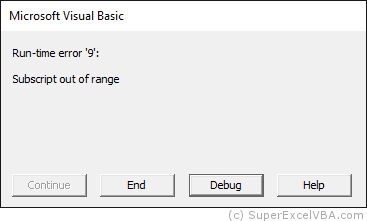# VBA Array

As seen so far, a variable can store only one value. However, through arrays it is possible to store more than one value in a variable.

Arrays are extremely useful in loops, as they make the code smaller and they make the code execute faster than with a variable per data.

## Arrays

Arrays are declared in a slight different way than variables.

    Dim AnArray(2) As String
'Dim DisplayName ([array size]) TheDataType


The Arrays have index to locate where data is being stored.

    Dim AnArray (10) As Integer

AnArray (3) = 5
AnArray (4) = 7

MsgBox "Index 3 stores" & AnArray (3) 'Index 3 stores 5
MsgBox "Index 4 stores" & AnArray (4) 'Index 4 stores 7


By default, VBA considers 0 as the first index element, so the above example has 3 elements:

    Dim AnArray(2) As String
AnArray(0) = "One"
AnArray(1) = "Two"
AnArray(2) = "Three"


Another way to declare a 3 elements array is to define the beginning and end in the index declaration.

    Dim AnArray(1 To 3) As String 'AnArray(Beginning to End)


In case above, we have a slightly different index arrangement, beginning at (1) and ending at (3), according to statement.

    AnArray(1) = "One"
AnArray(2) = "Two"
AnArray(3) = "Three"


If you are trying to associate a value with an undefined index (not declared in the array declaration), you will get an execution error:

    Dim AnArray(3) As String

AnArray(5) = "Five"The table below summarizes the ways to declare an array of defined size:

Declaration Element Nº1 Element Nº2 Element Nº3
Dim ArrName(2)
ArrName(0) ArrName(1) ArrName(2)
Dim ArrName(1 To 3)
ArrName(1) ArrName(2) ArrName(3)

It is not possible to create arrays starting with negative indexes.

    Dim AnArray(-3) 'This will generate an error
Dim AnArray(-1 To 3) 'This will generate an error


## Matrices

By declaring an array that has at least two dimensions (separated by commas) we create a matrix:

    Dim AMatrix(3,5) as String


In this case, this matrix has the first dimension of size 4 (from 0 to 3) and the second dimension of size 6 (from 0 to 5) thus totaling 24 elements ($4 \times 6=24$).

We assign values to this matrix in a way similar to arrays, specifying the element in which we want to assign a value:

    Dim AMatrix(3,5) as String
AMatrix(1,2) = "First with Second"


Similarly to arrays, we can specify the indexes with beginning and end.

    Dim AMatrix(1 To 4, 1 To 6) as String


We can also merge the declaration:

    Dim AMatrix(1 To 4, 5) as String


To copy and paste values from a matrix to a worksheet and vice versa, or to transpose the matrix, see the topic : Matrix Range.

## Dynamic Sizes Arrays

If you do not know the size of your array, you can choose to use dynamic arrays, where the limits are defined after the declaration.

Version ReDim

    Dim AnArray() As Integer
'... codes
ReDim AnArray(7) 'Resizes AnArray() to AnArray(7)


Version Variant/Array

    Dim AnArray As Variant
AnArray = Array("Super", "Excel", "VBA")
'The Array function transforms the arguments into elements of an array


Remember that in this case AnArray(0) will have the value of "Super", AnArray(1) will have the value of "Excel" and so on.

By using the Array function, you can transform elements of different data types into an array:

    AnArray = Array("Super", "Excel", 7)


However this practice is not recommended in order to avoid posterior data type errors, for example in loops.